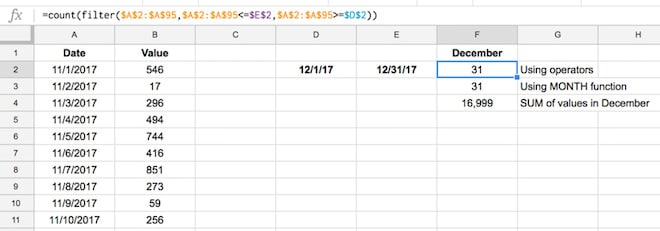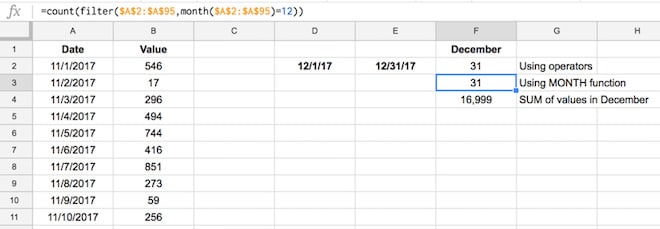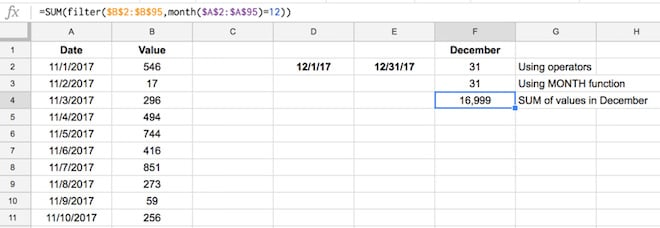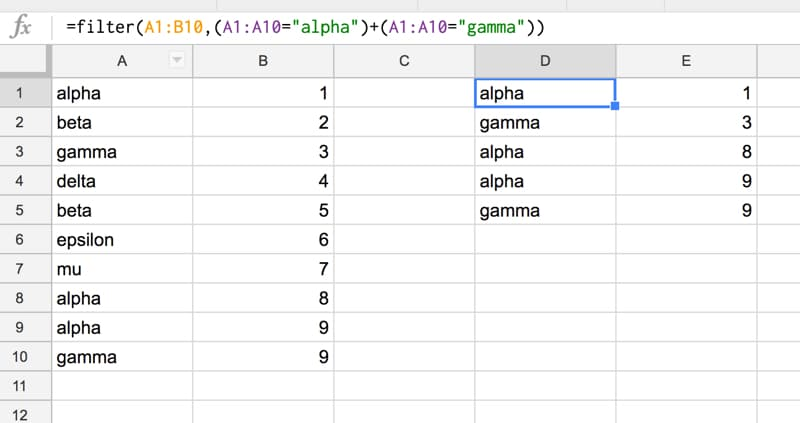If you’ve read my getting-started article on the Filter function in Google Sheets, you’ll know that it’s a very powerful function when working with data in Google Sheets.

In this post, I want to share a few more advanced filter options, such as working with dates and using OR logic.

### Example 1: Using Reference cells and Aggregation

Here’s an example using the FILTER function to aggregate (count and sum) how many values lie between two dates. I’m using the fictitious data in the template sheet with a date and associated value for that day.

In this scenario, we want to count how many values occur in December 2017 and then sum their value, using the FILTER function:To count the values, first put 12/1/17 and 12/31/17 into two adjacent cells and then try this formula in the next cell, as follows:

`=COUNT( FILTER( \$A\$2:\$A\$95, \$A\$2:\$A\$95 <= \$E\$2, \$A\$2:\$A\$95 >= \$D\$2 ))`

### Example 2: Use nested functions inside the Filter function

We can make the above formula more concise by nesting the MONTH function inside of the Filter function:

`=COUNT(FILTER(\$A\$2:\$A\$95, MONTH(\$A\$2:\$A\$95)=12))`

and it will give you the same answer of 31:### How do I sum filtered cells in Google Sheets?

Use this formula:

`=SUM(FILTER(\$B\$2:\$B\$95, MONTH(\$A\$2:\$A\$95)=12))`

We’ve done two things:

i) changed the filter range to column B (but not the test conditions!) to get the values, and

ii) wrapped the filter function in a SUM formula instead of a count.

The result is now:### How can I use OR logic inside an advanced filter?

If you know the trick for this advanced filter, this is quite easy to do, but if you don’t, well…

…then it’s easy to get lost in nested OR functions inside of FILTER functions and end up stranded in the land of errors.In this example I have 10 rows of data in columns A and B, as follows, and I want to retrieve all of the “alpha” and “gamma” values.

Rather than running two separate filter formulas, and then combining the results, we can use one formula that gives us the desired output:

`=FILTER(A1:B10,(A1:A10="alpha")+(A1:A10="gamma"))`

We use a “+” instead of a comma to handle the OR logic.

### How does this advanced filter formula work?

Consider a basic FILTER function like this one, for example, which returns only data with “alpha” in column A:

`=FILTER(A1:B10,A1:A10="alpha")`

Now, let’s see how the advanced filter works.

The next step is to return all the data that matches “alpha” or matches “gamma”.

We do this by using a “+” as the boolean operator OR inside the second argument of the FILTER function, as follows:

`=FILTER(A1:B10,(A1:A10="alpha")+(A1:A10="gamma"))`

Now, anytime either condition of the filter is satisfied, in other words an “alpha” or a “gamma” in column A is present, then that row of data is returned in our output filtered dataset.

## Other resources

Have you found a use for this advanced FILTER + OR formula? Any questions? Let me know in the comments below!

1.Dan says:

Hi, phenomenal walkthrough! If I wanted to refer to a cell instead typing in the text how would I go about doing that? For instance instead of “alpha” what if you wanted it to reference A1 where the “alpha” data resided? I keep getting formula parse errors. Thank you much!

1.Ben says:

Hey Dan,

You can just change the formula to this:

`=filter(A5:B14,(A5:A14=A1)+(A5:A14=B1))`

where I have my data range in A5:B14, and my filter values in A1 and B1 (e.g. alpha or beta or gamma etc.)

Hope that helps!

Cheers,
Ben

2.Matt Secrist says:

Ben, thanks for this. Have a question for you. What if my OR conditions were more complicated? Like, what if my filter is for multiple conditions to be met for both sides of the “or”? I’ll try to explain what I mean. For instance, what if I want it to filter something based on whether A1:A10=”alpha”, B1:B10>5, or if A1:A10=”gamma”, B1:B105)+(A1:A10=”gamma”,B1:B10<5)) but that doesn't seem to be working for me. Any help?

1.Lisa says:

Did you ever figure this out? Asking for a friend 🙂

1.Rohan Carter says:

Use one condition argument for FILTER and *. To create more complex conditions, bracket as necessary.

The article didn’t explain exactly how the + works. Whenever Google Sheets expects a boolean, it is looking for a TRUE or FALSE value. 0 evaluates to FALSE and all other values evaluate to TRUE.

Using +’s between n operators gives you 0 if none are true, n when n are true (more importantly, not 0).

In a similar vein, using the * to add an AND condition works because it multiples your current result by either 0 (if the condition is false) or something bigger than 0 (if true). i.e. If your condition is not true, then your entire evaluation will end up FALSE.

Here’s an example using the article’s data to get all values that are alpha or gamma, but only those greater than 4:

=FILTER(A1:B10, ( (A1:A10=”alpha”) + (A1:A10=”gamma”) ) * (B1:B10>4) )

1.Ben says:

2.ibrahim says:

thank you so so much for the explaination

3.Darshil R Desai says:

Amazing! Really helps me build out a simple database from a master sheet using different conditions.

4.Peter says:

Hi Ben

Is it possible to set a “named range” as the filter criteria?

Let’s say I want to use the “OR” function shown in the example, but I have a list of 50 items I want to include in my filter. Do I need to build up 50 “OR” segments in the formula, or is there a way to link to a named range?

1.Ben says:

Hey Peter,

You can use a named range in a filter, but it won’t solve this OR issue.

I’ve not found a way to succinctly do this, other than building up the 50 OR segments. You can use a formula like this to build the 50 segments as text, which you can then copy paste into your filter formula:

`=ArrayFormula(mid(concatenate("+(A2:A11="""&transpose(unique(\$D\$2:\$D))&""")"),2,len(concatenate("+(A2:A11="""&transpose(unique(\$D\$2:\$D))&""")"))-13))`

I’ve not found a way to embed this in the filter function however.

If I find anything, I’ll post it in the article here.

Cheers,
Ben

5.Daniel says:

Hi, Thanks for all the help, my problem it’s a little bit different, I want to syncronize a Google form with a Google Sheet, I know it is easy, but also, I want to create another sheet that automatically update the Date in one cell, for example: you send “Alpha” with the google form with a date, then you send “Beta”, Beta has his own cell, but, how do I create a Filter that update the date when you send “Beta” or “Apha”? Thanks for all the help!!

1.Ariel says:

Great post Ben
Thanks a lot 😁😁

6.Markus says:

Greetings,

Can the OR filter be used in a filter view, which would result in your original data set (A1:B10) being reduced to where the only viewable/visible data then becomes what you have in D1:E5 (but would appear in A1:B5)? Hopefully I’ve accurately described what I’m trying to accomplish. I have data that will potentially encompass A4:AP401 in a my spreadsheet (as more an more data gets added). I wish to filter out that data which is entered by specific people (name of person who entered the data appears in column C). That is why I am trying to create a filter view. One option is to create it via Value, whereby the people’s names can be checked off.
The issue I have come across is that method not cumulative. What I mean is that if the data set is to be populated by Jon, Joe, Bill, Dave, Lisa and Ted, and I wish to only see the data entered by Jon and Joe, I have to create a filter view and then manually go in can check off their respective names. However, if Jon enters data, but Joe has not yet, only Jon appears as an option to check off. Which means I have to wait until Joe enters data, go back into the filter view, and check off his name, so then his subsequent data will appear. What I want to do is set up a filter view ahead of time so that whenever Jon and Joe enter data, they automatically appear in the view (without having to go back in and manipulate the filter view). Thanks for your time.

7.North says:

Thank you for this guide! It seems to be exactly what I’m looking for, however I’m running into a bit of trouble. I’ve tried these formulas:

=filter(Input!C8:O,(MATCH(Input!I8:I,INDIRECT(D8),0))+(MATCH(Input!I8:I,SPLIT(C8,”,”),0)))
^ gives me #N/A “No matches are found in FILTER evaluation”, though there should be.

=filter(Input!C8:O,(Input!I8:I=INDIRECT(D8))+(Input!I8:I=SPLIT(C8,”,”)))
^ gives me #VALUE! “FILTER range must be a single row or a single column.”

=filter(Input!C8:O,OR(MATCH(Input!I8:I,INDIRECT(D8),0)),MATCH(Input!I8:I,SPLIT(C8,”,”),0))
^ gives me #N/A “FILTER has mismatched range sizes. Expected row count: 994, column count: 1. Actual row count: 1, column count: 1.” (The Input sheet has 1001 rows, with data starting at row 8. The sheet with this search function is 1000 rows, and the cell where this function is being tested is C18.)

Input!C8:O is my spread of data, Input!I8:I is a column listing specific colors. INDIRECT(D8) refers to a named range; the cell D8 has a Data Validation list of text options, and when one is selected (ex. “Greens”), INDIRECT fits it into the function to draw data from that named range (ex. Green, Emerald, Seafoam). C8 is a cell with multiple text criteria separated by commas, ex. “Red,White,Blue”. I do intend to add more criteria to search other columns, but for now the goal with this formula is to look at column I on the Input sheet and find all rows that have Red, White, Blue, *and* all Greens in column I and return that data.

The first formula seems most promising as I can get it working exactly as intended if only one of the criteria is present (and either set of criteria works), but once I try to search for both at once, it’s errors everywhere. QUERY would probably be a better option for searching with this criteria, but it seems the data shown by QUERY doesn’t carry over hyperlinks, so I have to stick with FILTER. I can’t seem to find what I need just by Googling, so if you know of a fix or any suggestions I could try, it would be greatly appreciated!

8.Cody says:

Hi Ben,

Thank you for the article. I have followed your example however it still is coming back with an error. My formula is

`"=FILTER('House Buying Analysis'!A:V,'House Buying Analysis'!(D:D="Richmond") + (D:D="Burnley"))"`

The error is “Formula Parse Error”.

I was just wondering if you had any idea why it is not working? Thank you in advance!

1.Ben says:

Hey Cody,

The issue is with how you’ve written the ranges in the filter criteria, because they’re presumably in a different tab than your formula. Try this version:

Cheers,
Ben

9.Lauren says:

HI dan-
This is very helpful. I’m having trouble however trying to find the mode of one column based on filters of other columns.
For example I need to find the mode of Column N, based on the contents of column X, N and B.
B=1
N=Stores
X=discounted
Y=values of discount (for which i need the mode.)

Do you have any suggestions? Thank you so much in advance 🙂

10.SHAHIRA says:

I HAVE A TWO SHEETS ONE IS CUSTOMER SHEET AND OTHER IS CODE WISE PRICE DETAILS .I WANT TO SEARCH RECORD BASED ON A CUSTOMER AND CODE PLZ GIVE DETAILS OF FUNCTION.

11.John says:

With regard to using a named range or indeed CSV text as filter values rather than hard coding them as above I enclose three slightly different variations on the OR filter idea without the OR!, rather using MATCH instead which would seem to be the more obvious approach since it is a matching exercise.
The suggested solution may be found at
Regards.
John B

12.Jesse says:

I’m curious how one would do this on an importrange logic in another workbook like this:
=FILTER(IMPORTRANGE(“sheetkey”, “Checks”), INDEX(IMPORTRANGE(“sheetkey”, “Checks”),0,8)=”ABC”)

1.Celia Bolton says:

I have the same question.

13.Peter says:

Hi Ben,

The data is filtering in perfectly except that once it is filtered, I am having a problem filtering the data A-Z or Z-A.

For example, I am using a master spreadsheet with data headers “Name, Venue, Time, Date, Review.” From that master spread sheet I am filtering data by Name into individual sheets. Example sheet 1 is “Joe Smith” and all “Joe Smith” data is filtered from master sheet to sheet 1. Once all that data is filtered in, I am having difficulty filtering “Venue” from A-Z or Z-A. I have set up the filter on sheet 1 but it does not perform the function when commanded. Any help???

1.Ben says:

From what I can figure out from my experimenting, the sorting (A-Z/Z-A) is linked to the sorting in the master sheet, so if you switch the sorting in the master sheet to “Venue”, you should find that this also happens in Sheet 1.

14.Nelson says:

Ben Collin,

Congratulations for you expetise, dedication e colaboration with the community.
In due course, I will post an issue where your experience could be of extreme value.

15.PNM says:

Hi Ben,

Is there a walk around where we multiple conditions are optional and not always selected in drop down.
Example for filtering student data
Data validation A1 is Age, B1 Class, C1 City, D1 Gender
=FILTER(Data!A:D,(Data!A:A=A1)+(Data!B:B=B1)+(Data!C:C=C1)+(Data!D:D=D1))
can we get results even when all 4 options are not selected matching any 1 or all can filter results

16.Eric says:

Is it possible to combine filter and sort. I would need to first filter the data and in a second step sort it according to a specific column.

17.Guy says:

Hi Ben,
Is there a way to use the filter function results as a reference for a data validation list of values ?

18.George says:

Does someone have a formula I can apply to Google Sheets that filters data in one sheet based on the column values of another?

19.Niaz says:

I’m getting “That function isn’t valid” when trying to execute anything similar to =count(filter(range,range=”criteria”))

20.Vici says:

Hi – I’m trying to come up with a filter that copies a row from main sheet (ALL DATA) if the date (timestamp) in Column A is 30 days or older and if the status in Column P is “Archived”. I’ve come up with the following, but it doesn’t work:

=filter(ALLDATA!A:S, “select P, where (dateDiff(now(), A) > 30) and P!=’Archived'”)

Can anyone assist?

21.Josef says:

Hi,
I would like to ask for help with following formula creation.
On the tab “Dashboard” I have 3 data validation lists to get averages based on the selection. I created there option for “ALL” however I am not able to figure it out the formula to show me results (averages from all) when selecting “ALL”. I tried to use OR…but no result.
Thanks for help.

22.Tod Poirier says:

Hi, I would like to ask for help with a filter function I’m having trouble with. I don’t know if it’s directly possible, but I would like to create a sheet that compiles rows of data from 2 other sheets. I have the functioned created for one sheet: =filter(Programs!A:O,Programs!\$E:\$E=”Architecture”)
but none of the variations I’ve tried will allow me to add a 2nd sheet to the results, an example of one attempt that doesn’t work: =filter(Programs!A:O,Programs!\$E:\$E=”Art”, [Series!A:O,Series!\$E:\$E=”Art”])
Thanks!

1.Russel says:

Tod,
I’m trying to do the same exact thing. Did you figure this out?

23.Andrew Clark says:

Hi Ben

I’ve tried using filters in column Q of that tab, to move or copy the role into the corresponding tab, like this: https://docs.google.com/spreadsheets/d/1ImF1rOZYL3tiLDFYzb_KgDt_mzSJFDWOJLkmreLzrYI/edit#gid=1572613331 – but can’t get it to work.

Any ideas?

Cheers
Andrew

24.Mayank says:

is there a way to use like condition in custom filter? for example i want to filter a column where the text like ‘%text%’, all above example s seems to be for absolute value

25.Stas says:

Thank you for this guide, Ben! It seems to be really close to what I’m looking for, however I’m running into a bit of trouble implementing a line break insertion while trying to join the output of my formula into a single cell as a multiline list of values.
My formula
=IF(A2=””;””;TRANSPOSE(SORT(FILTER(‘Sheet1′!\$B\$2:\$B;’Sheet1′!\$AA\$2:\$AA=\$A2;’Sheet1’!\$AB\$2:\$AB=\$B2))))
extracts a few values and puts them into a few columns of a line but I need them combined into a single cell with CHAR(10) for line break.

26.Ngat says:

Give me a help. I want to filter results which appear in column A or column B . For example , I have sheet Data . I want to find all results have text Ex in column A or Ex in column B and have text 1, 2 or 3 in column C . If I use this formula: FILTER(Data!A:C,(Data!A:A=Ex)+(Data!B:B=Ex),(Data!C:C=1)+(Data!C:C=2)+(Data!C:C=3)), is it ok??? I think it is logical but it didn’t bring right results 🙁

27.Kharri Jamison-Crawford says:

I am having trouble with filtering in my sheet. I would like to have the sheet show me the rowes that have phone numbers that begin with only certain area codes. I can do it in excel but want to know how to do this in my GoogleSheets page. I used this formula
=FILTER(A1:L155,C2″216″,C2″440″,C2″330″)
and was retured an #ERROR! (Formula parse error.)
Can you assist me with this?

28.Gary Miller says:

I have a problem with FILTER, in that it does not seem to work when the filtered data is pulled in with IMPORTRANGE, e.g. =Filter(IMPORTRANGE(“https://docs.google.com/spreadsheets/d/abcdgobbledegookxyz”,”Table!A2:S170″),P2:P170=”Stuff”) always says Error – No matches are found in filter evaluation.

Why doesn’t this work?

1.Ben says:

Gary – You have to give permission to the importrange before you can nest it in other formulas. So try importrange on its own, allow it, then wrap with filter. Should work!

Cheers,
Ben

29.AD:AM says:

Is there a way to use the filter formula to:

1) Compare Column A to Column B
2) Remember the unique values in Col B that do not exist in Col A
3) Compare the unique values found in Col B and then compare it to Col C
4) Output any duplicates find in Col C to Col D

I tried using variations of =MATCH function but it only checks if there is a matching pair.

The formula I used:
=FILTER(trim(B2:B),trim(A2:A<2), match(trim(B2:B),trim(C2:C)))

For example, if Col A has the value "test" a single time, but Col B has "test" entered twice, it will only recognize the first instance of "test" in Col B as a match, not the second instance (which makes sense, I guess).

Also, the other problem with my formula is that if there is a number value like "1", it will return "1" as a result even if it's not a duplicate. I suspect because I'm calculating match(A2:A<2), which "1" certainly qualifies.

30.Dexter says:

Hi Dan,

I’m having a hard time on this. For the examples above, they only have 2 criteria to filter. What if they have 3 criteria to filter? Can’t seem to figure it out.
A B C
Alex Break 1 16
Ben Break 2 17
Dan Meal 62
Alex Meal 60
Ben Break 1 15
Dan Break 2 17

Say threshold for Break 1 and 2 is 15 and for meal, 60. How can I filter that it would only show the Break >15 and meals >60.

I’ve used:
=filter(A:C,(B:B=Break 1)+(B:B=Break 2),C:C>15)

I don’t know where to insert the filter criteria for meal >60.

Hope to hear from you soon.

31.Susan says:

Not sure if this will help anyone… But I finally figured out how to get INDIRECT(), ARRAYFORMULA(), and FILTER(), working together to reference values from another sheet.
I like to use a counta() formula to get the exact row count because too often I have had formulas break because someone put data in the wrong place, And due to the fact that I have gotten into combining the formulas with apps script, I found this very useful in getting rowData.
My Formula:
=ArrayFormula( IF( Indirect(“‘Daily sheet'”&”!”&”A4:A”&G1)””,ArrayFormula(‘Daily sheet’!\$B\$2-‘Daily sheet’!\$A\$4:\$A),””) )

Where G1 holds a COUNTA() formula to get a flexible row count from the daily sheet, tucked inside an array formula that only grabs the rows of data and not the blank spaces.
Hope this will help someone else.

32.Brian says:

This is really great. But I only need a few columns from a very wide sheet in my output. Is there a way to include non-contiguous columns in the filter output? something like filter((a1:e3,n1:p3),condition)? Thanks.

33.Linda B says:

Hi
I am trying to multiply the result of a cell but only want to multiply the 2 decimal points that are showing. Rounded amount.
A1= 3.657 cell is formatted to show 2 decimal points A1=3.66
I want my result to equal \$10.00 * 3.66 = \$36.60
The result I am getting is \$10.00*3.657 = \$36.57
Can someone assist me?
Thank you

1.Matthew says:

Hey Linda B,
I believe I can help with this. If you are wanting to leave your original A1 cell alone then this is what I would do. In the cell that you are multiplying by 10.00 in try the formula “=ROUND(A1,2)*10.00”
This here should return your desired \$36.60. That is provided that you format the cell to be currency.
I hope this helps!,
Matthew

34.bhatti says:

Hi,

Is there any way to get multiple rows from a google sheets.
For Example if I want to pull data from a sheet where I have 2 or 3 entries with same name. How I can pull data of all 2 or 3 names please.

Thank you.

35.Matthew says:

Hi,

So I have sort of a complicated question that I have been working on for awhile now. So what I am trying to do is return a specific value when a list of dates reaches a date that is greater than or equal to a specified date. In other words I want this certain calculated value to appear once a certain date is reached or a date past the specified date is input into the row of cells that track the dates.
My dates start in A2 and the specified date in this case is F5 which is equal to 1/15/2020. The value to return let’s say is the formula H2*12.5 if the date is reached or passed. Otherwise I would like the cell to remain empty. So far I have tried this formula:

“=IF(IFNA(FILTER(\$A\$2:\$A\$35, \$A\$2:\$A\$35>=F5),””)>=F5, H2*12.5,””)” however this is not properly tracking correctly I would really love some help with this

36.Halis Batmaz says:

hi, how can I translate the vba advanced filter code I have mentioned below in the google apps scrip.

——————-
Sub Makro2()

37.AexV says: